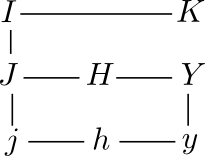# Bessel functions in SciPy

Python’ SciPy library has many functions for evaluating Bessel functions. All are contained in the `scipy.special` subpackage.

There are several varieties of Bessel functions, all closely related as summarized in the diagram below.Each letter is the conventional symbol for a family of Bessel functions. The lines between letters indicates a simple relationship between function families. For more on the definition of each function and how they are related, see Relations between Bessel functions.

Each family of Bessel functions is indexed by a parameter called the order. By convention, the order is denoted n when integer-valued and ν (Greek nu) when more generally real-valued. These notes will focus on the general case of real orders.

The table below summarizes the correspondence between Bessel functions and their SciPy implementations.

Math SciPy
Jν `jv`
Yν `yv`
Iν `iv`
Kν `kv`
Hν(1) `hankel1`
Hν(2) `hankel2`
jν `sph_jn`
yν `sph_yn`

In general SciPy names use a Roman letter `v` at the end of a function corresponding a mathematical function with a Greek ν subscript. Similarly, SciPy uses `n` for integer-order specializations that would be denoted with a subscript n. The Python functions `jv`, `yv`, and `kv` have integer specializations `jn`, `yn`, and `kn`.

All SciPy functions in the table take two arguments. The first is the order and the second is the parameter. For example, Jv(z) is evaluated using `jn(v, z)`.

The Hankel functions H(1) and H(2) are implemented in the SciPy functions `hankel1` and `hankel2`. SciPy does not contain functions for implementing the spherical Hankel functions h(1) and h(2), though these functions can be easily be computed in terms of the spherical Bessel functions. See relationship here.

The spherical Bessel functions jn and yn have integer order and are implemented in the SciPy functions `sph_jn` and `sph_yn`. These SciPy functions are unusual in two ways: they return values of the functions up to and including the specified order, and they return derivative values as well. For example, `sph_jn(2, 3.4)` returns a pair of arrays. The first array contains j0(3.4), j1(3.4), and j2(3.4) and the second array contains j0(3.4), j1(3.4), and j2(3.4).

SciPy includes optimized specializations for Bessel functions of orders 0 and 1. The functions `j0` and `j1` are optimized versions of `jv` with first argument 0 and 1 respectively. There are analogous optimized versions of `yv`, `iv`, and `kv`.

SciPy contains many other functions related to Bessel functions: exponentially scaled Bessel functions, derivatives and integrals of Bessel functions, and zeros of Bessel functions. It also contains implementations of Ricatti-Bessel functions and Struve functions, functions closely related to the Bessel functions discussed here.

Related page: Gamma and related functions in SciPy.